##### How Does the CEILING() Function Work in PostgreSQL?

PostgreSQL supports various mathematical functions that help us round a numeric value up to the specified decimal places. CEILING() is one such function that rounds the given decimal number up to the nearest integer. It is the equivalent of the CEIL() function, as both these functions round up the given to the nearest integer. It is equally effective for rounding negative numbers.

This post explains the various use cases of the CEILING() function along with suitable examples.

How Does the CEILING() Function Work in Postgres?

In Postgres, CEILING() is a built-in math function that accepts a numeric or double precision value and converts it into the nearest integer toward the positive infinity. Here is the basic syntax:

`CEILING(num);`

Where num represents any positive or negative numeric or double precision number. The return type of the CEILING() function depends on the data type of the input value.

Example 1: Using CEILING() Function With Positive Value

In the following example, the CEILING function is utilized on a positive value to get a rounded value:

`SELECT CEILING(250.77);`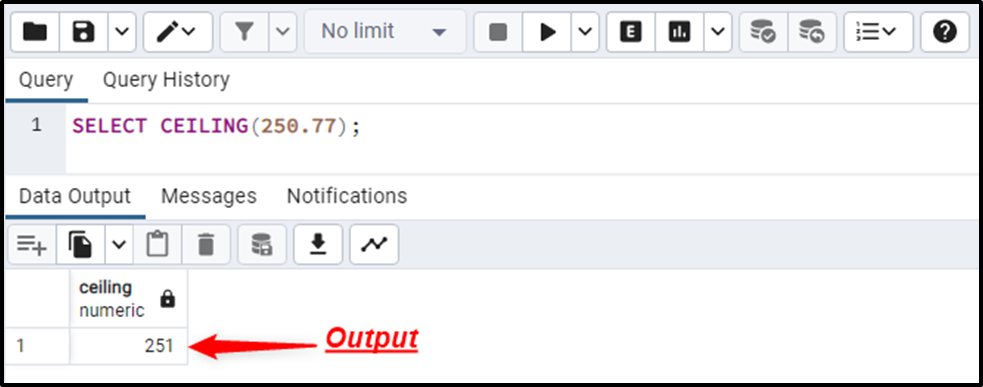The output signifies that the input numeric value has been rounded up to the nearest integer.

Example 2: Using CEILING() Function With Negative Value

In the following example, the CEILING function is utilized on a negative to get a rounded integer:

`SELECT CEILING(-250.77);`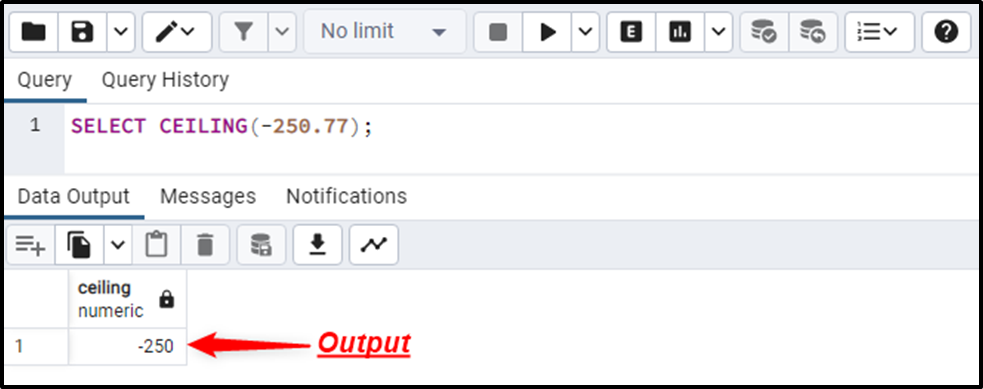The output verifies that the given numeric value has been rounded up to the nearest integer.

Example 3: CEILING() VS CEIL() VS FLOOR() VS ROUND() VS TRUNC()

In the following example, we will utilize the CEILING(), CEIL(), FLOOR(), ROUND(), and TRUNC() functions side by side:

```SELECT CEILING(272.77),
CEIL(272.77),
FLOOR(272.77),
ROUND(272.77),
TRUNC(272.77);```

Here in the above snippet:

- The CEILING() and CEIL() functions are used to round up the given numeric value to the nearest integer.
- The FLOOR() function is utilized to round down the input numeric value to the closest integer.
- The ROUND() function will round the given numeric value upward or downward based on the specified fractional part.
- The TRUNC() function will trim the fractional points and retrieve the integer value.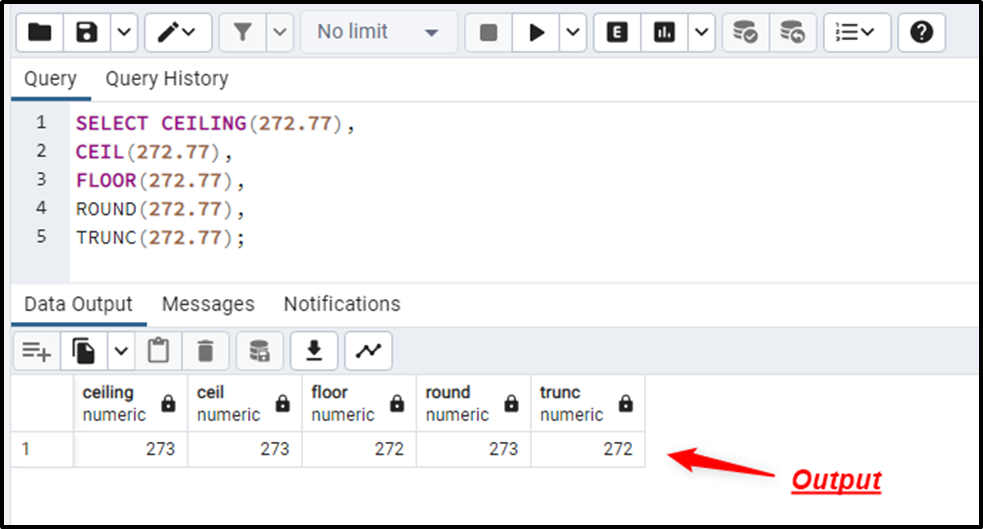The output demonstrates the difference between the CEILING(), CEIL(), FLOOR(), ROUND(), and TRUNC() functions.

Example 4: Using CEILING() Function on Table’s Data

In this example, we will utilize the “product_details” table that we have already created in our database:

`SELECT * FROM product_details;`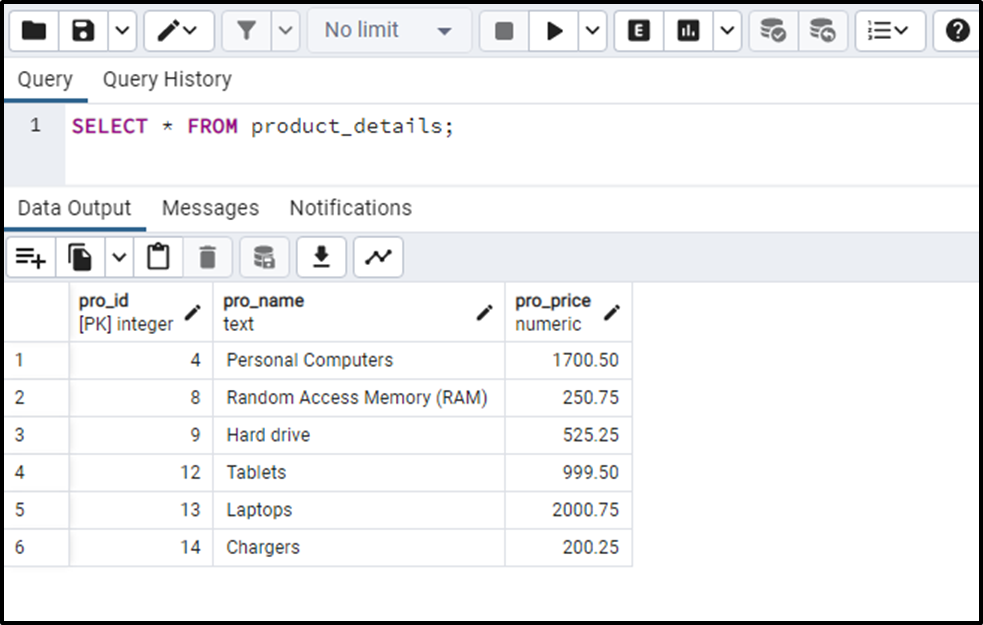Let’s utilize the CEILING() function to round the “pro_price” column up to the nearest integer:

```SELECT pro_name, pro_price, CEILING(pro_price)
FROM product_details;```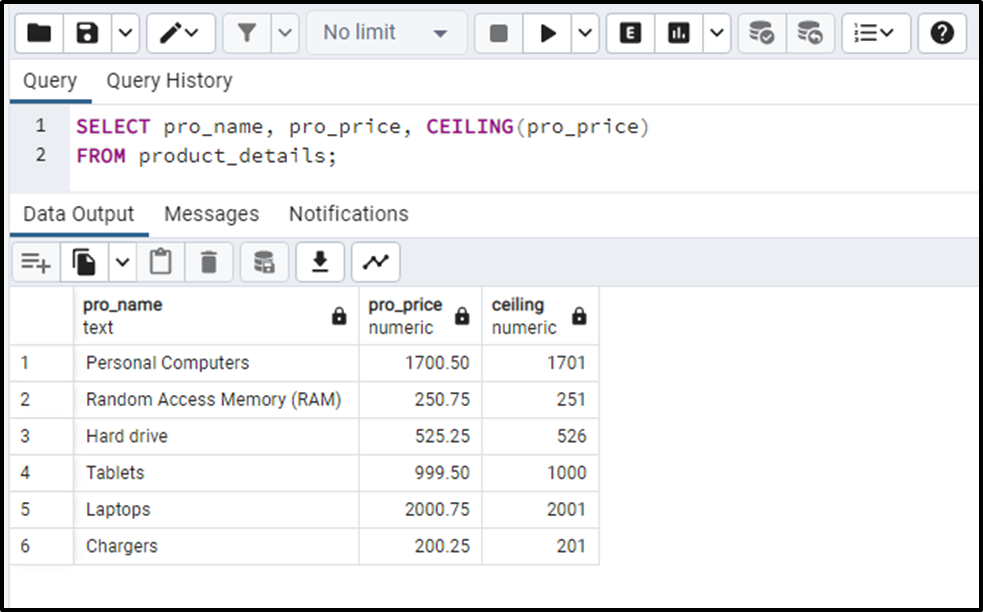The output shows that the CEILING() function successfully rounded the given values up to the nearest integers.

Conclusion

In Postgres, CEILING() is a built-in math function that accepts a numeric or double precision value and converts it into the nearest integer toward the positive infinity. It is equally effective for rounding negative numbers. It is the equivalent of the CEIL() function, as both these functions round up the given to the nearest integer. This post explained how the CEILING() function works in Postgres using appropriate examples.